What：什么是 table-driven？

// GetWeekDay returns the week day name of a week day index.
func GetWeekDay(index int) string {
if index == 0 {
return "Sunday"
}
if index == 1 {
return "Monday"
}
if index == 2 {
return "Tuesday"
}
if index == 3 {
return "Wednesday"
}
if index == 4 {
return "Thursday"
}
if index == 5 {
return "Friday"
}
if index == 6 {
return "Saturday"
}
return "Unknown"
}

// GetWeekDay returns the week day name of a week day index.
func GetWeekDay(index int) string {
if index < 0 || index > 6 {
return "Unknown"
}
weekDays := []string{"Sunday", "Monday", "Tuesday", "Wednesday", "Thursday", "Friday", "Saturday"}
return weekDays[index]
}

• 稳定的流程
• 定义测试用例
• 定义输入数据和期望的输出数据
• 跑测试用例，拿到实际输出
• 比较期望输出和实际输出
• 易变的数据
• 输入的数据
• 期望的输出数据

Why：为啥单测要 table-driven？

• 写得快：人类只需准备数据，无需构造流程。
• 可读性强：将数据构造成表，结构更清晰，一行一行的数据变化对比分明。
• 子测试用例互相独立：每条数据是表里的一行，被流程模板构造成一个独立的子测试用例。
• 可调试性强：因为每行数据被构造成子测试用例，可以单独跑、单独调试。
• 可扩展/可维护性强：改一个子测试用例，就是改表里的一行数据。

例子一：低质量单测之平铺多个 test case

// test case for index=0
func TestGetWeekDay_Sunday(t *testing.T) {
index := 0
want := "Sunday"
if got := GetWeekDay(index); got != want {
t.Errorf("GetWeekDay() = %v, want %v", got, want)
}
}

// test case for index=1
func TestGetWeekDay_Monday(t *testing.T) {
index := 1
want := "Monday"
if got := GetWeekDay(index); got != want {
t.Errorf("GetWeekDay() = %v, want %v", got, want)
}
}

...

例子二：低质量单测之平铺多个 subtest

func TestGetWeekDay(t *testing.T) {
// a subtest named "index=0"
t.Run("index=0", func(t *testing.T) {
index := 0
want := "Sunday"
if got := GetWeekDay(index); got != want {
t.Errorf("GetWeekDay() = %v, want %v", got, want)
}
})

// a subtest named "index=1"
t.Run("index=1", func(t *testing.T) {
index := 1
want := "Monday"
if got := GetWeekDay(index); got != want {
t.Errorf("GetWeekDay() = %v, want %v", got, want)
}
})

...

}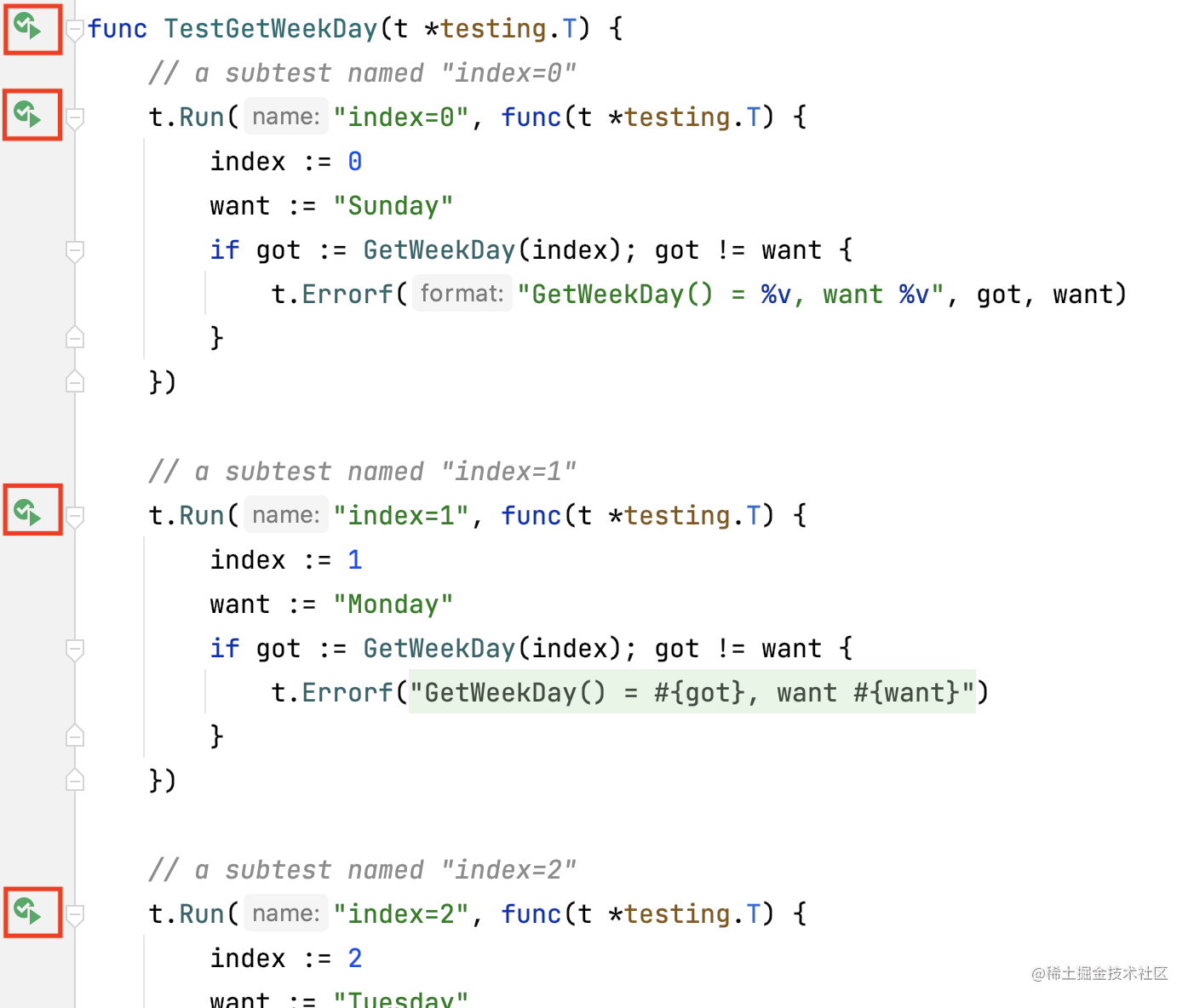go test 的 log，也支持结构化输出 subtest 运行结果：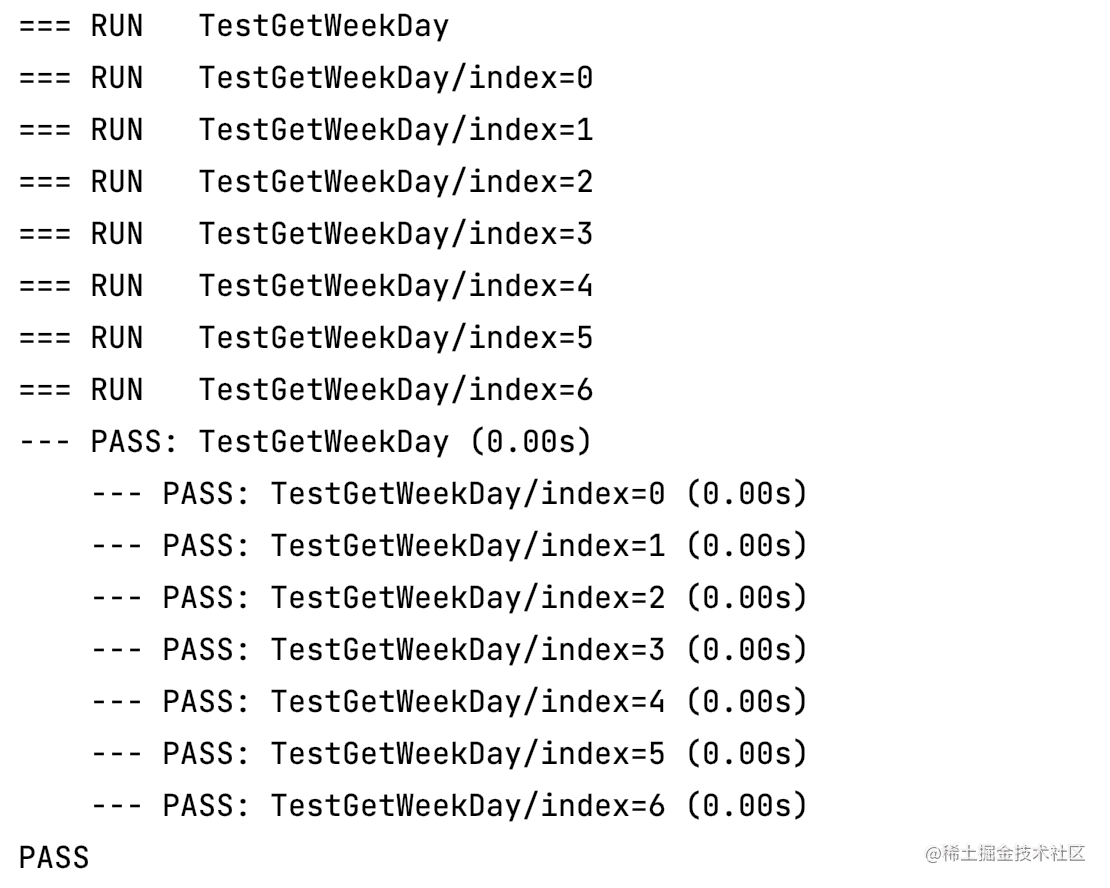例子三：高质量单测之 table-driven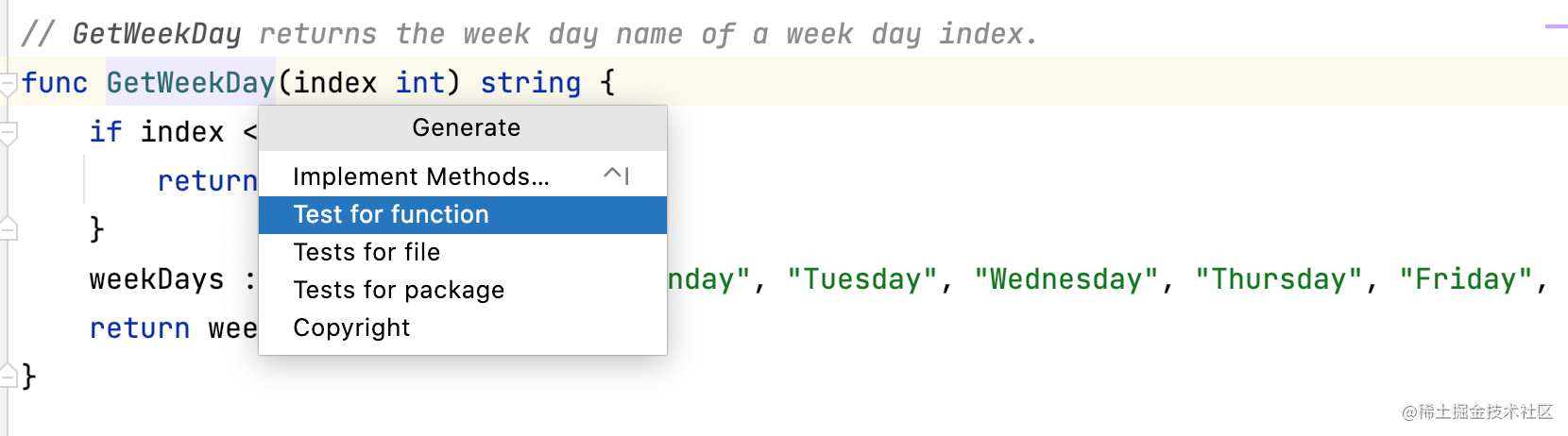GoLand 会自动生成如下模板，而我们只需填充红框部分，也即最核心的，用于驱动单测的数据表：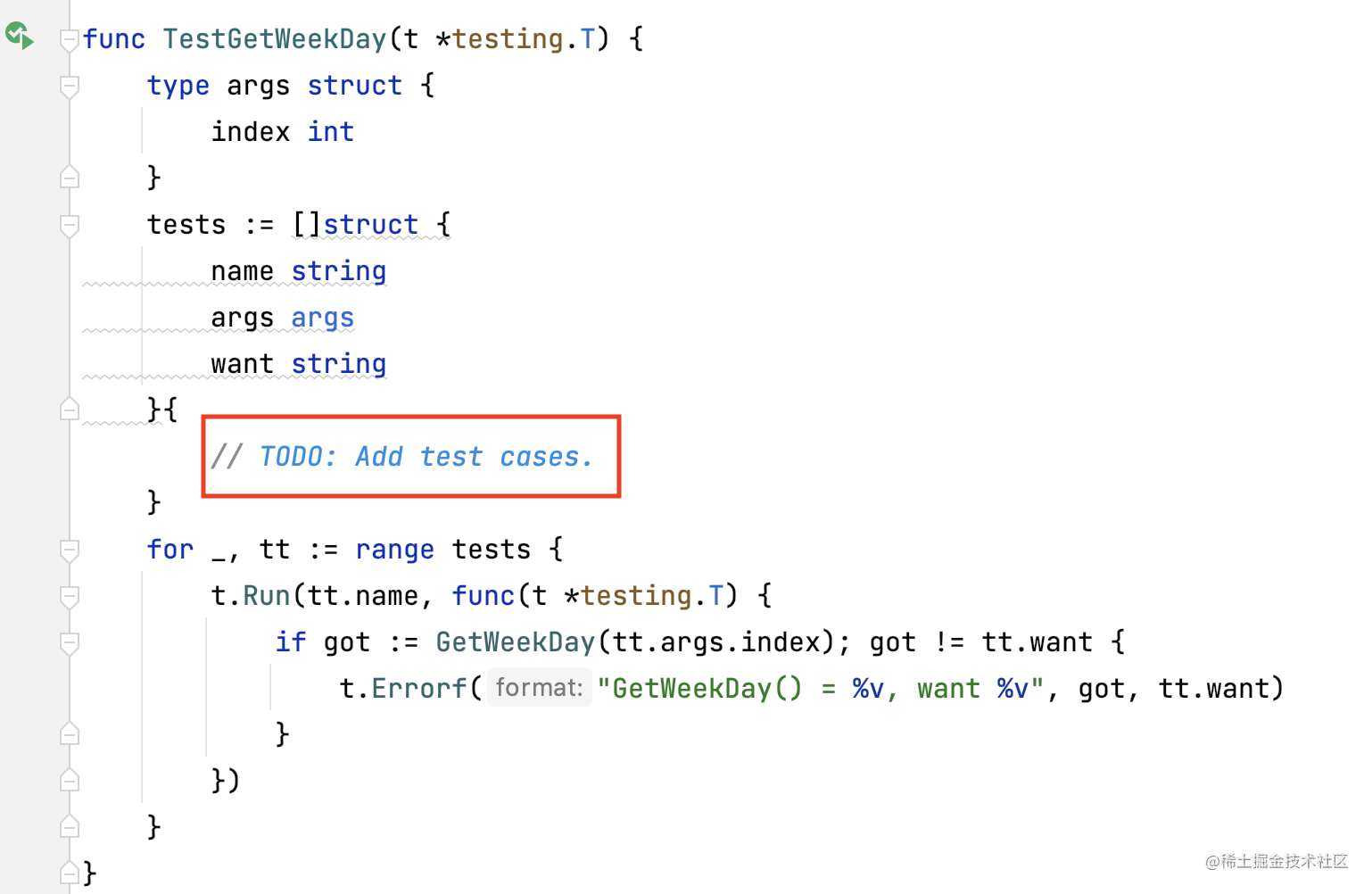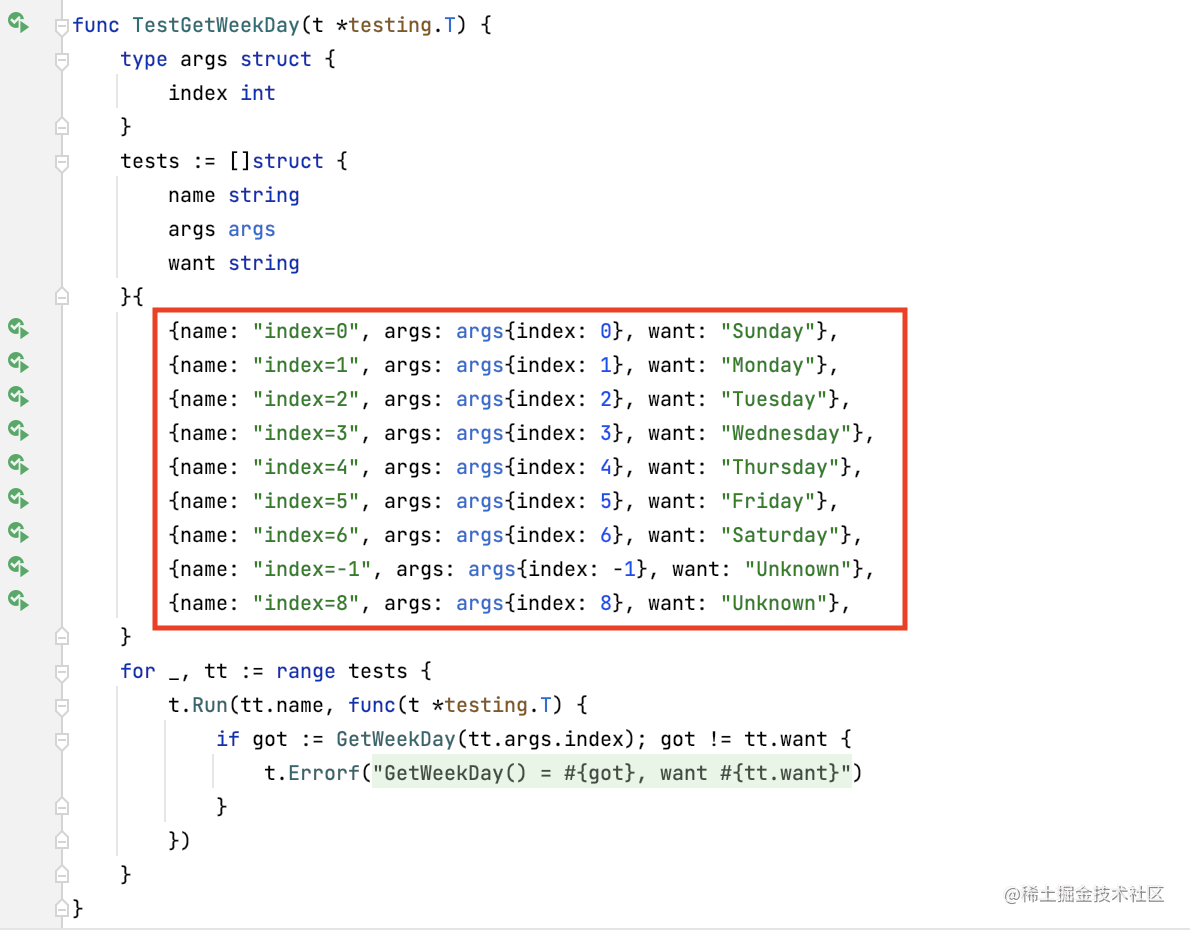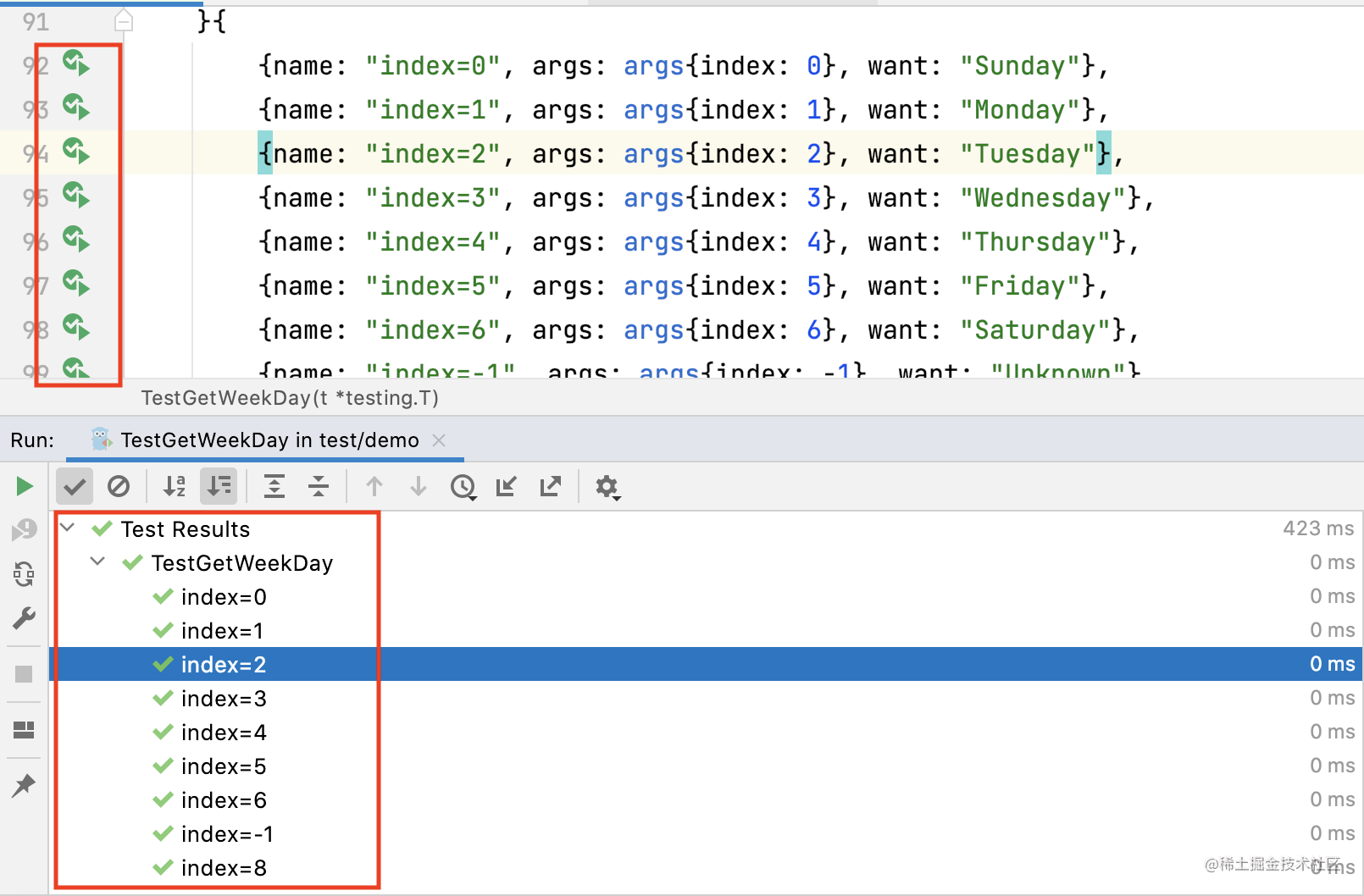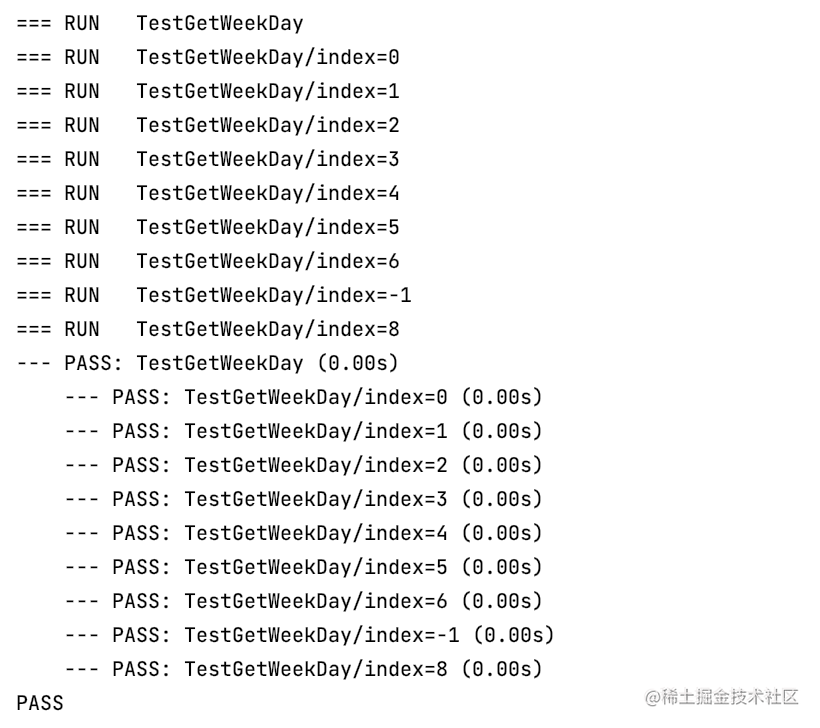How：怎么写 table-driven 单测？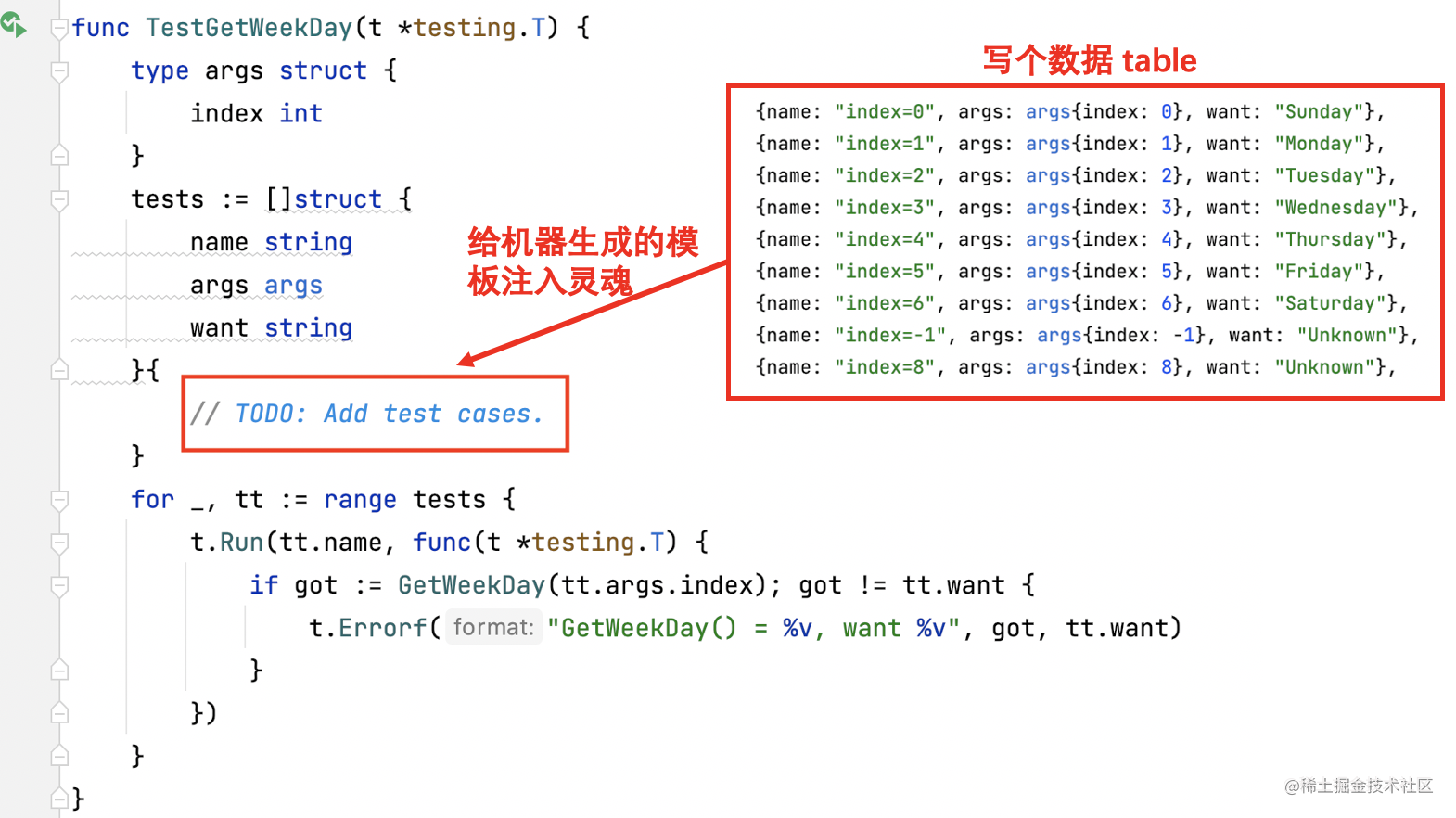func TestGetWeekDay(t *testing.T) {
type args struct {
index int
}
tests := []struct {
name string
args args
want string
}{
{name: "index=0", args: args{index: 0}, want: "Sunday"},
{name: "index=1", args: args{index: 1}, want: "Monday"},
{name: "index=2", args: args{index: 2}, want: "Tuesday"},
{name: "index=3", args: args{index: 3}, want: "Wednesday"},
{name: "index=4", args: args{index: 4}, want: "Thursday"},
{name: "index=5", args: args{index: 5}, want: "Friday"},
{name: "index=6", args: args{index: 6}, want: "Saturday"},
{name: "index=-1", args: args{index: -1}, want: "Unknown"},
{name: "index=8", args: args{index: 8}, want: "Unknown"},
}
for _, tt := range tests {
t.Run(tt.name, func(t *testing.T) {
if got := GetWeekDay(tt.args.index); got != tt.want {
t.Errorf("GetWeekDay() = %v, want %v", got, tt.want)
}
})
}
}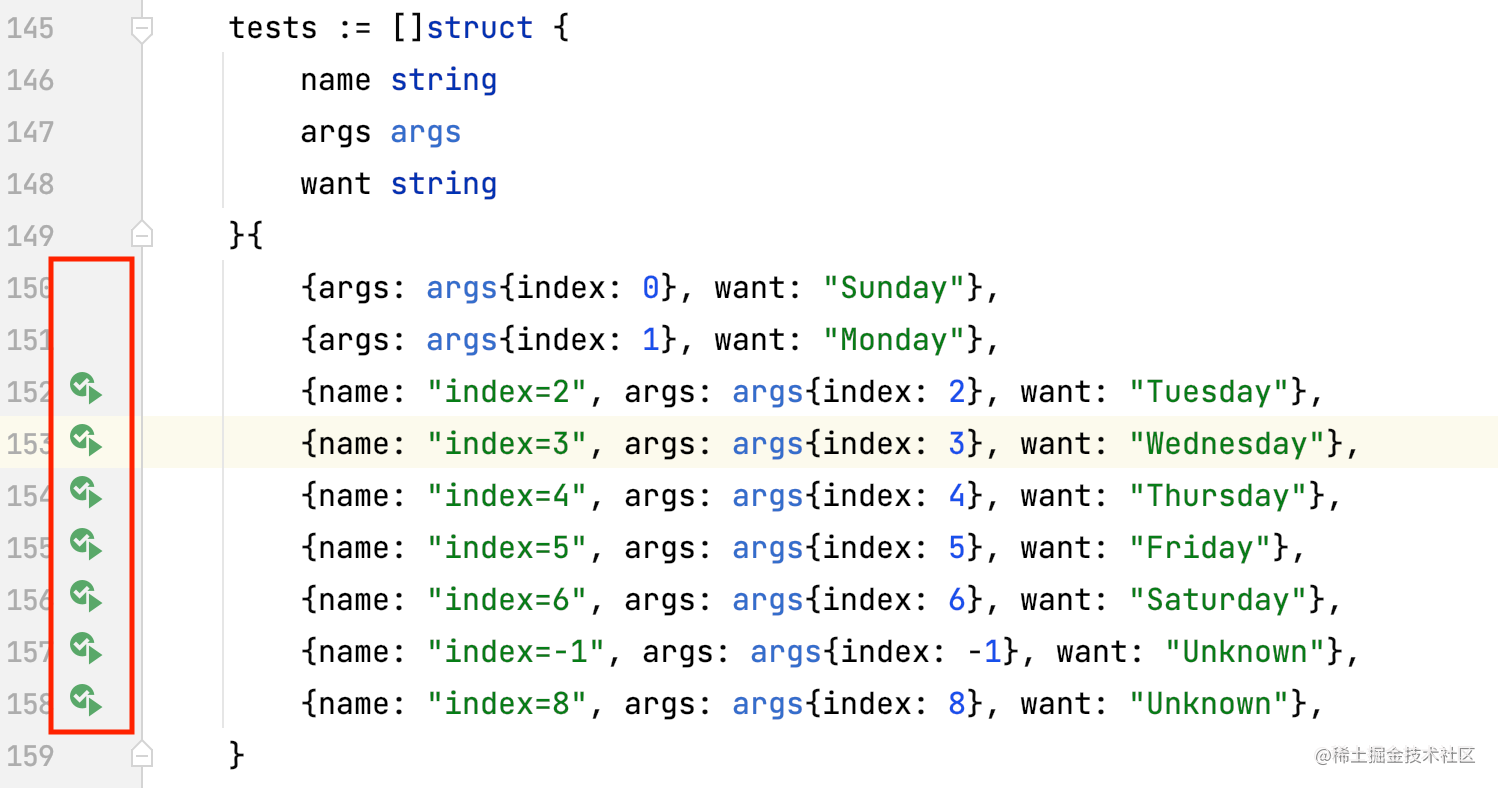高阶玩法

table-driven + parallel

for _, tt := range tests {
tt := tt // 新变量 tt
t.Run(tt.name, func (t *testing.T) {
t.Parallel() // 并行测试
t.Logf("name: %s; args: %d; want: %s", tt.name, tt.args.index, tt.want)
if got := GetWeekDay(tt.args.index); got != tt.want {
t.Errorf("GetWeekDay() = %v, want %v", got, tt.want)
}
})
}

1. for 循环迭代器的变量 tt，是被每次循环所共用的。也即，tt 一直是同一个 tt；每次循环只改变了 tt 的值，而地址和变量名一直没变。
2. 每个加了 t.Parallel 的 subtest，被传给自己的 go routine 后不会马上执行，而是会暂停，等待与其并行的所有 subtest 都初始化完成。
3. 那么，当 Go 调度器真正开始执行所有 subtest 的时候，外面的for循环已经跑完了；其迭代器变量 tt 的值，已经拿到了循环的最后一个值。
4. 于是，所有 subtest 的 go routine 都拿到了同一个 tt 值，也即循环的最后一个值。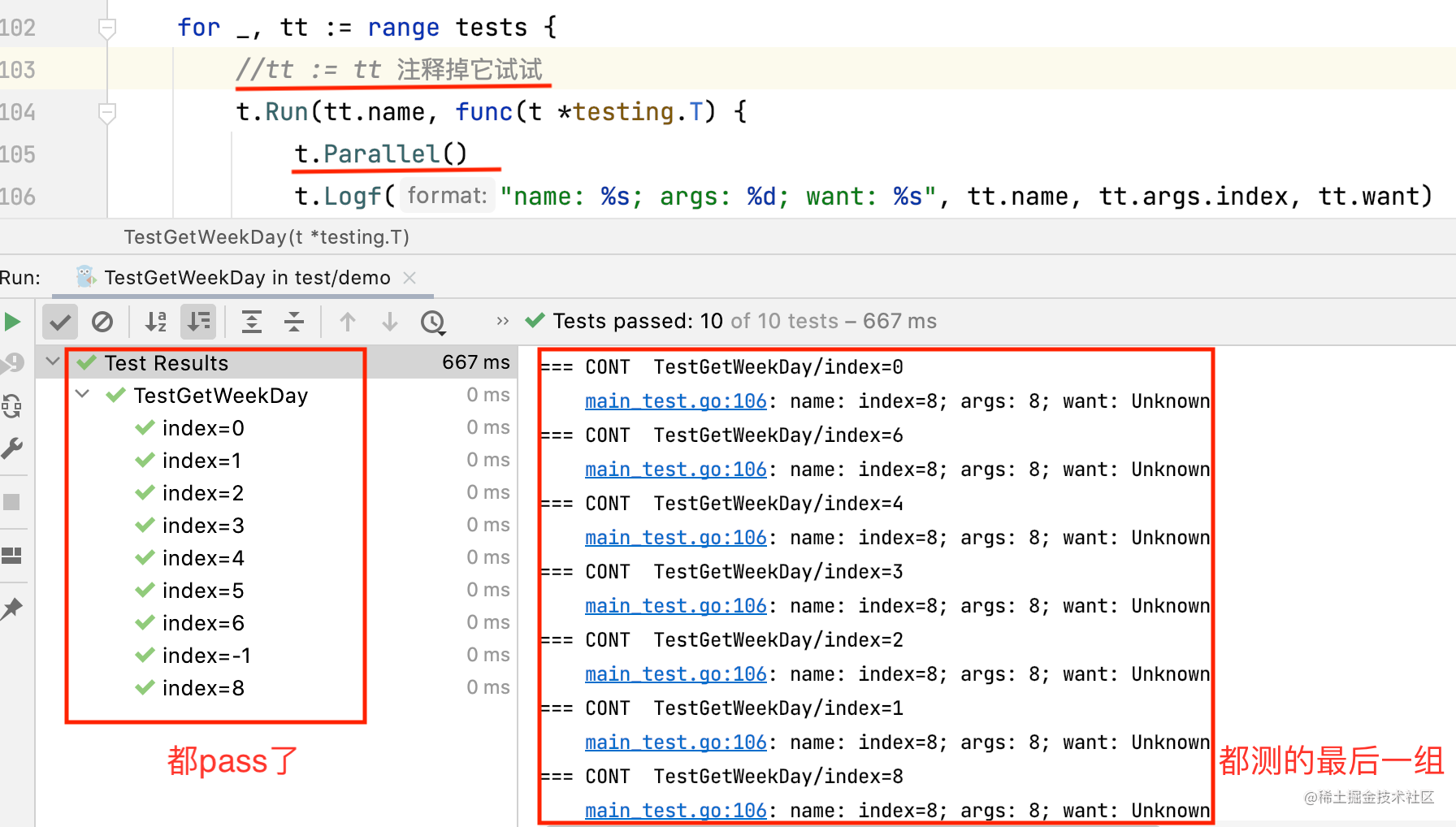table-driven + assert

Go 的标准库本身不提供断言，但我们可以借助 testify 测试库的 assert 子库，引入断言，使得代码更简洁、可读性更强。

if got != tt.want {
t.Errorf("GetWeekDay() = %v, want %v", got, tt.want)
}

assert.Equal(t, tt.want, got, "should be equal")

func TestGetWeekDay(t *testing.T) {
type args struct {
index int
}
tests := []struct {
name string
args args
want string
}{
{name: "index=0", args: args{index: 0}, want: "Sunday"},
{name: "index=1", args: args{index: 1}, want: "Monday"},
{name: "index=2", args: args{index: 2}, want: "Tuesday"},
{name: "index=3", args: args{index: 3}, want: "Wednesday"},
{name: "index=4", args: args{index: 4}, want: "Thursday"},
{name: "index=5", args: args{index: 5}, want: "Friday"},
{name: "index=6", args: args{index: 6}, want: "Saturday"},
{name: "index=-1", args: args{index: -1}, want: "Unknown"},
{name: "index=8", args: args{index: 8}, want: "Unknown"},
}
for _, tt := range tests {
t.Run(tt.name, func(t *testing.T) {
got := GetWeekDay(tt.args.index)
assert.Equal(t, tt.want, got, "should be equal")
})
}
}

{name: "index=0", args: args{index: 0}, want: "NotSunday"},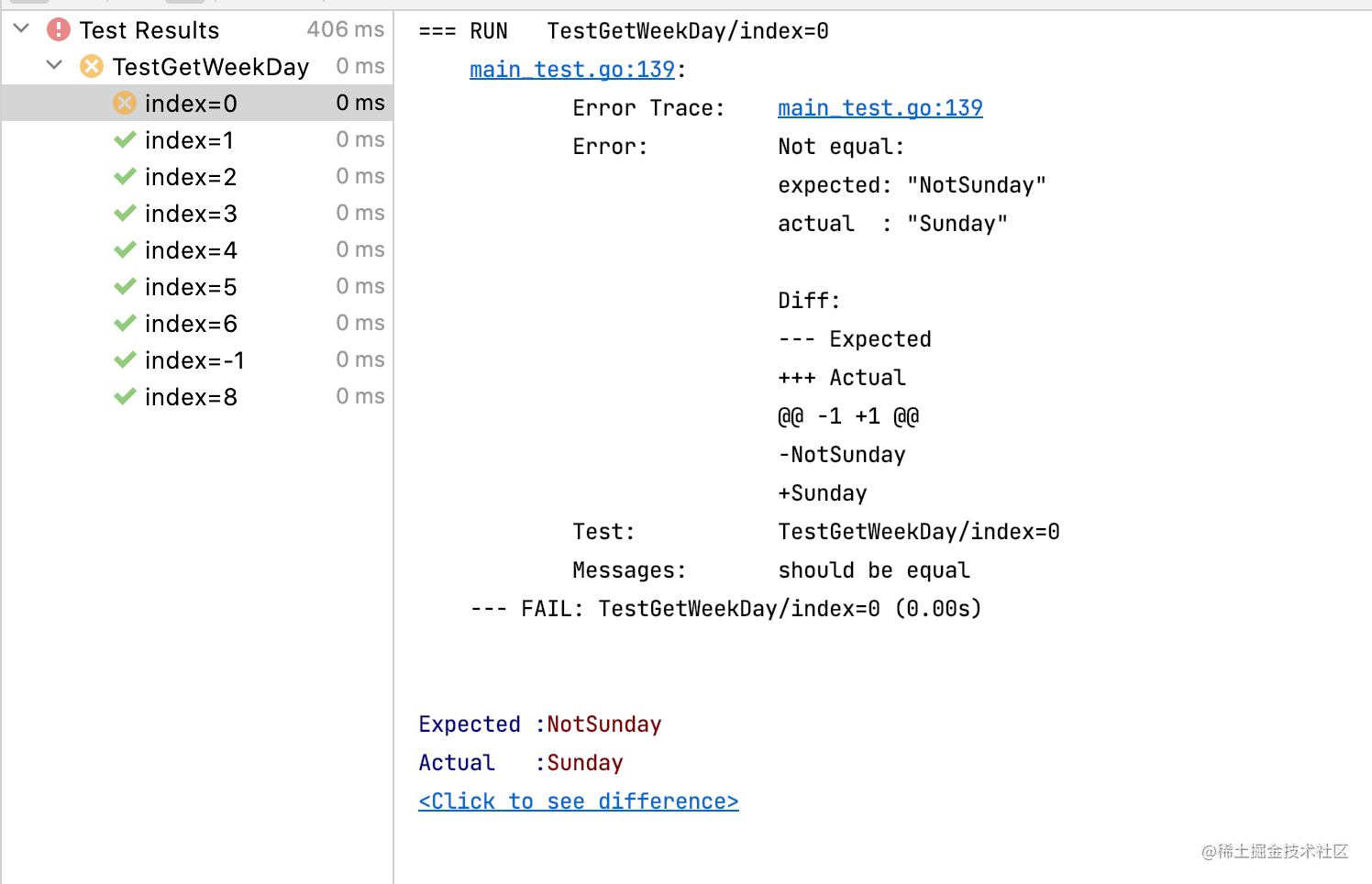func TestGetWeekDay(t *testing.T) {
type args struct {
index int
}
tests := []struct {
name   string
args   args
assert func(got string)
}{
{
name: "index=0",
args: args{index: 0},
assert: func(got string) {
assert.Equal(t, "Sunday", got, "should be equal")
}},
{
name: "index=1",
args: args{index: 1},
assert: func(got string) {
assert.Equal(t, "Monday", got, "should be equal")
}},
}
for _, tt := range tests {
t.Run(tt.name, func(t *testing.T) {
got := GetWeekDay(tt.args.index)
if tt.assert != nil {
tt.assert(got)
}
})
}
}

table-driven + mock

package main

type WeekDayService interface {
GetWeekDay(int) string
}

type WeekDayClient struct {
svc WeekDayService
}

func (c *WeekDayClient) GetWeekDay(index int) string {
return c.svc.GetWeekDay(index)
}

mockgen -source=weekday_srv.go -destination=weekday_srv_mock.go -package=main

package main

import (
"github.com/golang/mock/gomock"
"github.com/stretchr/testify/assert"
"testing"
)

func TestWeekDayClient_GetWeekDay(t *testing.T) {
// dependency fields
type fields struct {
svc *MockWeekDayService
}
// input args
type args struct {
index int
}
// tests
tests := []struct {
name    string
fields  fields
args    args
prepare func(f *fields)
assert  func(got string)
}{
{
name: "index=0",
args: args{index: 0},
prepare: func(f *fields) {
f.svc.EXPECT().GetWeekDay(gomock.Any()).Return("Sunday")
},
assert: func(got string) {
assert.Equal(t, "Sunday", got, "should be equal")
}},
{
name: "index=1",
args: args{index: 1},
prepare: func(f *fields) {
f.svc.EXPECT().GetWeekDay(gomock.Any()).Return("Monday")
},
assert: func(got string) {
assert.Equal(t, "Monday", got, "should be equal")
}},
}
for _, tt := range tests {
t.Run(tt.name, func(t *testing.T) {
// arrange
ctrl := gomock.NewController(t)
defer ctrl.Finish()
f := fields{
svc: NewMockWeekDayService(ctrl),
}
if tt.prepare != nil {
tt.prepare(&f)
}

// act
c := &WeekDayClient{
svc: f.svc,
}
got := c.GetWeekDay(tt.args.index)

// assert
if tt.assert != nil {
tt.assert(got)
}
})
}
}

1. fields 是 WeekDayClient struct 里的字段，为了 mock，单测时将里面的外部依赖 svc 的原本类型 WeekDayService，替换为 mockgen 生成的 MockWeekDayService。
2. 在每个 subtest 数据里，加一个 func 类型的 prepare 字段，可将 fields 作为入参，在 prepare 时对 fields.svc 的多种行为进行 mock。
3. 在每个 t.Run 的准备阶段，创建 mock 控制器、用该控制器创建 mock 对象、调 prepare 对 mock 对象做行为注入、最后将该 mock 对象作为接口的实现，供 WeekDayClient 作为外部依赖使用。

自定义模板

func Test\$NAME\$(t *testing.T) {
// dependency fields
type fields struct {
}
// input args
type args struct {
}
// tests
tests := []struct {
name    string
fields  fields
args    args
prepare func(f *fields)
assert  func(got string)
}{
}
for _, tt := range tests {
tt := tt
t.Run(tt.name, func(t *testing.T) {
// run in parallel
t.Parallel()

// arrange
ctrl := gomock.NewController(t)
defer ctrl.Finish()
f := fields{}
if tt.prepare != nil {
tt.prepare(&f)
}

// act

// assert
if tt.assert != nil {
tt.assert(\$GOT\$)
}
})
}
}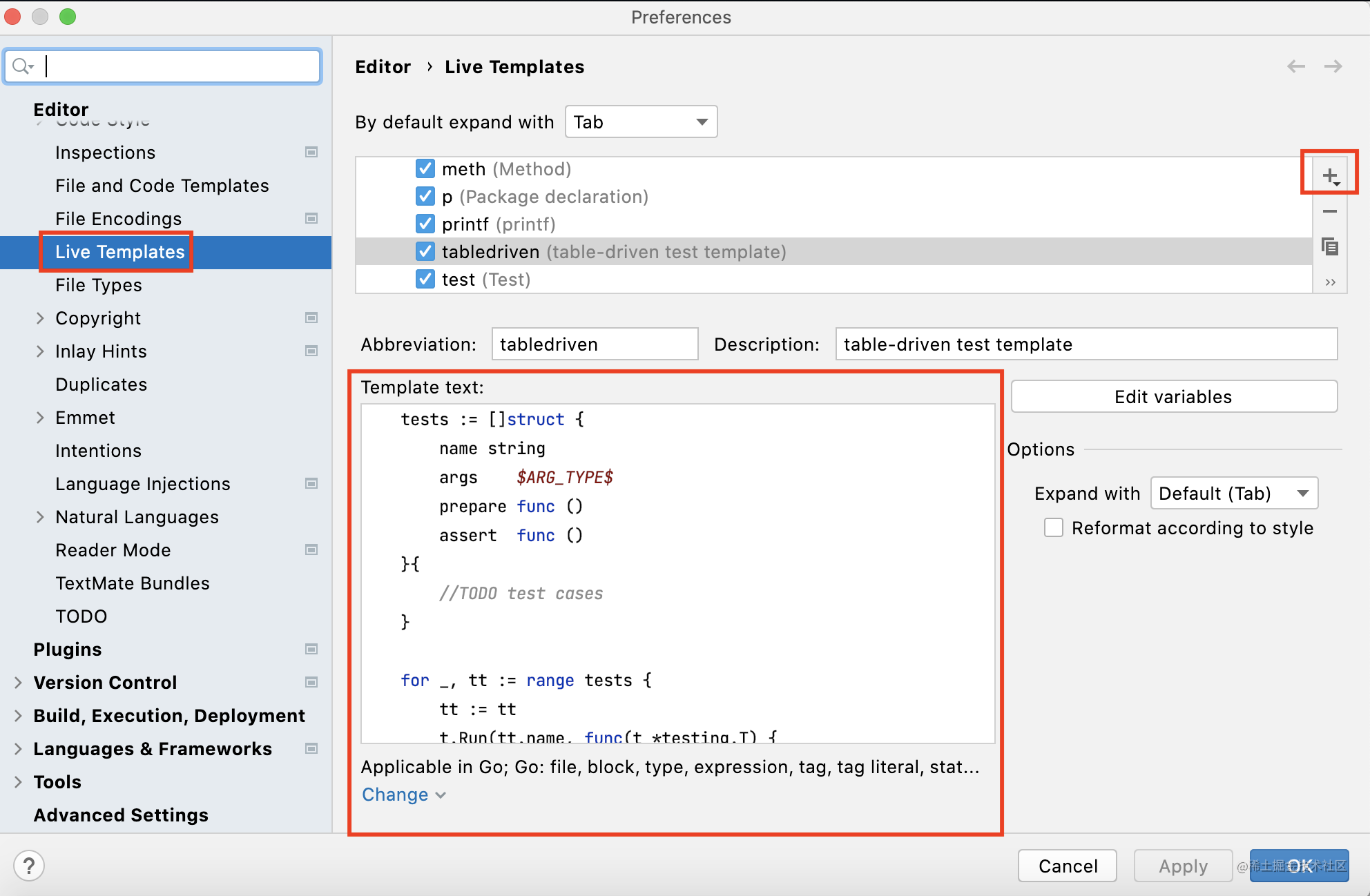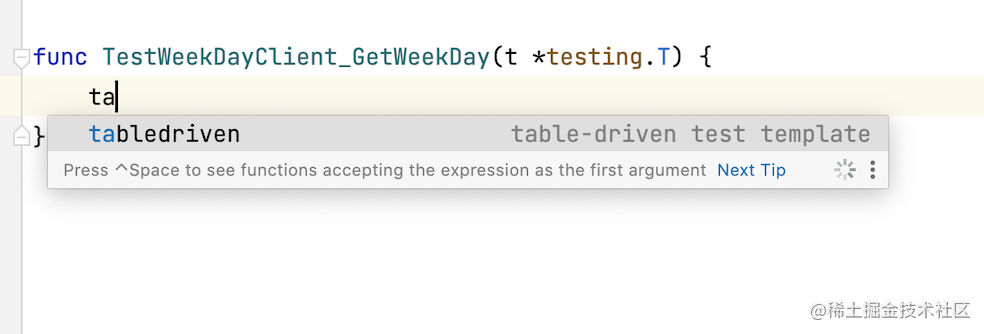❤️ 网络一线牵，

❤️ 珍惜这段缘。

❤️ 不妨点个赞，

❤️ 给您拜早年！